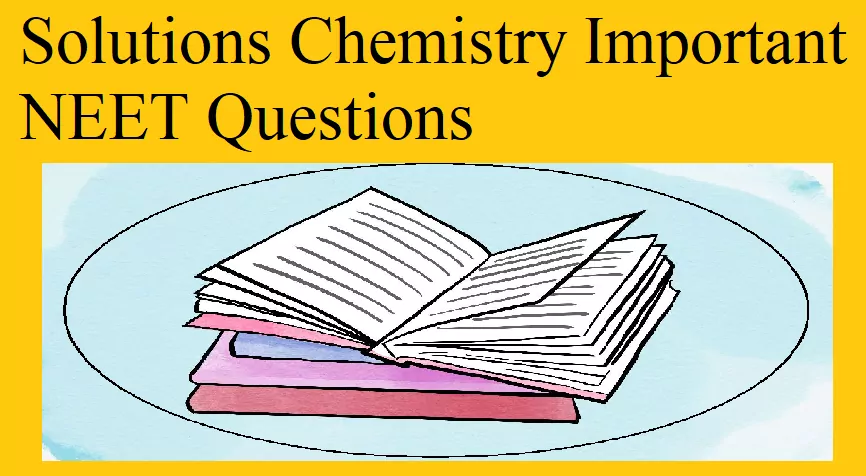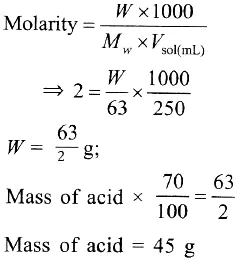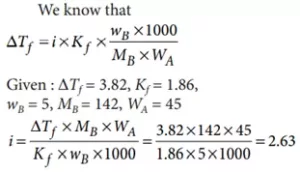# Solutions Chemistry Important NEET QuestionsSolutions Chemistry Important NEET Questions

Que 1. How many grams of concentrated nitric acid solution should be used to prepare 250 mL of 2.0 M HNO3? The concentrated acid is 70% HNO3.
(a) 70.0 g conc. HNO3
(b) 54.0 g conc. HNO3
(c) 45.0 g conc. HNO3
(d) 90.0 g conc. HNO3
Ans 1. (c)
Explanation:Que 2. In water-saturated air, the mole fraction of water vapour is 0.02. If the total pressure of the saturated air is 1.2 atm, the partial pressure of dry air is
(a) 1.18 atm                         (b) 1.76 atm
(c) 1.176 atm                       (d) 0.98 atm.
Ans 2.  (b)
Explanation: pwater vapour = xwater vapour × Ptotal
= 0.02 × 1.2 = 0.024 atm
Ptotal = pwater vapour + pdry air
1.2 = 0.024 + pdry air
pdry air = 1.2 – 0.024 = 1.176 atm
Partial vapour pressure is directly proportional to mole fraction, p α x.

Que 3. The mixture which shows a positive deviation from Raoult’s law is
(a) ethanol + acetone                                       (b) benzene + toluene
(c) acetone + chloroform                                  (d) chloroethane + bromoethane.
Ans 3. (b)
Explanation: A mixture of ethanol and acetone shows a positive deviation from Raoult’s law.
In pure ethanol, molecules are hydrogen-bonded. On adding acetone, its molecules get in between the host molecules and break some of the hydrogen bonds between them. Due to the weakening of interactions, the solution shows a positive deviation from Raoult’s law.

Solutions Chemistry Important NEET Questions

Que 4. Which of the following statements is correct regarding a solution of two components A and B exhibiting positive deviation from ideal behaviour?
(a) Intermolecular attractive forces between A-A and B-B are stronger than those between A-B.
(b) ∆mix H = 0 at constant T and P.
(c) ∆mix V = 0 at constant T and P.
(d) Intermolecular attractive forces between A-A and B-B are equal to those between A-B.
Ans 4. (a)
Explanation: In case of positive deviation from Raoult’s law, A-B interactions are weaker than those between A-A or B-B, i.e., in this case, the intermolecular attractive forces between the solute-solvent molecules are weaker than those between the solute-solute and solvent-solvent molecules. This means that in such a solution, molecules of A (or B) will find it easier to escape than in a pure state. This will increase the vapour pressure and result in a positive deviation.

Que 5. The freezing point depression constant (Kf) of benzene is 5.12 K kg mol-1. The freezing point depression for the solution of molality 0.078 m containing a non-electrolyte solute in benzene is (rounded off up to two decimal places).
(a) 0.20 K                               (b) 0.80 K                                (c) 0.40 K                   (d) 0.60 K
Ans 5. (c)
Explanation: Given Kf = 5.12 K kg mol-1, m = 0.078 m
ΔTf = Kf × m = 5.12 × 0.078 = 0.39936 ≈ 0.40 K

Que 6. A solution of sucrose (molar mass = 342 g mol-1) has been prepared by dissolving 68.5 g of sucrose in 1000 g of water. The freezing point of the solution obtained will be (Kf for water = 1.86 K kg mol-1).
(a)  – 0.372 °C                                (b) – 0.520 °C                  (c)  + 0.372 °C             (d) – 0.570 °C
Ans 6. (a)
Explanation:

Solutions Chemistry Important NEET Questions

Que 7. The van’t Hoff factor (i) for a dilute aqueous solution of the strong electrolyte barium hydroxide is
(a) 0                             (b) 1                            (c) 2                          (d) 3
Ans 7. (b)
Explanation: Being a strong electrolyte, Ba(OH)2 undergoes 100% dissociation in a dilute aqueous solution, Ba(OH)2 → Ba2+ + 2 OH
Thus, van’t Hoff factor i = 3.

Que 8. The van’t Hoff factor i for a compound that undergoes dissociation in one solvent and association in another solvent is respectively
(a) less than one and greater than one
(b) less than one and less than one
(c) greater than one and less than one
(d) greater than one and greater than one.
Ans 8. (c).
Explanation: From the value of van’t Hoff factor i it is possible to determine the degree of dissociation or association. In the case of dissociation, i is greater than 1 and in the case of an association, i is less than 1.

Que 9. The freezing point depression constant for water is –1.86 °C m-1. If 5.00 g Na2SO4 is dissolved in 45.0 g H2O, the freezing point is changed by –3.82 °C. Calculate the van’t Hoff factor for Na2SO4.
(a) 2.05                                (b) 2.63             (c) 3.11                                   (d) 0.381
Ans 9. (b)Solutions Chemistry Important NEET Questions

Que 10. Blood cells retain their normal shape in solution which are:
(a) hypotonic to blood                        (b) isotonic to blood
(c) hypertonic to blood                       (d) equinormal to blood.
Ans 10. (b)  Blood cells neither swell nor shrink in an isotonic solution. The solutions having the same osmotic pressure are called isotonic solutions.

Que 11. Which of the following aqueous solution has a minimum freezing point?
(a) 0.01 m NaCl                    (b) 0.005 m C2H5OH     (c) 0.005 m MgI2         (d) 0.005 m MgSO4
Ans 11. (a) Here, ΔTf = i × Kf × m
van’t Hoff factor, i = 2 for NaCl, so conc. = 0.02, which is maximum in the present case.
Hence, ΔTf is maximum or the freezing point is minimum in 0.01 m NaCl.

Que 12. Which one is a colligative property?
(a) Boiling point                                 (b) Vapour pressure
(c) Osmotic pressure                          (d) Freezing point
Ans 12. (c) Osmotic pressure.

Solutions Chemistry Important NEET Questions